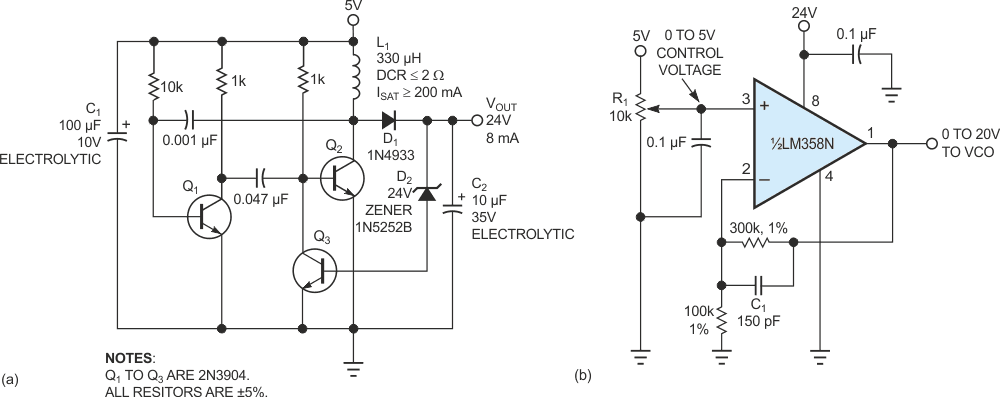# Low-Cost Switcher Converts 5 to 24V

## Texas Instruments LM358-N

The low-cost, three-transistor boost switching regulator in Figure 1a is a modified astable multivibrator comprising Q1, Q2, and L1, which substitutes as a load for Q2. At the full output power of 200 mW, the oscillation frequency is approximately 60 kHz. The efficiency is 65% with VOUT equal to 24 V and sourcing 8 mA.Figure 1. A simple three-transistor switching regulator (a) supplies 24 V and 8 mA. The circuit can help provide a 0 to 20 V VCO tuning voltage from a 0 to 5V control voltage (b).

When the base of Q2 is high, energy stores in L1’s magnetic field. When the circuit drives the base of Q2 low, the induced voltage from L1’s magnetic field collapses to add with the supply voltage. This voltage spike charges C2 through D1. When the accumulated charge in C2 results in a voltage equal to the zener voltage of D2 plus 0.6 V, Q3 pulls Q2’s base to ground, decreasing the amount of time Q2 is on in subsequent oscillations and thereby decreasing the energy transferred to C2. This feedback through D2 regulates the output voltage to 24.6 V±the tolerance of D2. To change the output voltage of the circuit, simply change the zener voltage of D2. Many VCOs require tuning voltages as high as 20 V, and you can use this switching regulator to generate a 0 to 20 V tuning voltage from a 0 to 5 V control voltage (Figure 1b). The circuit configures one-half an LM358N as a noninverting amplifier with a gain of 4. C1 eliminates gain for the noise generated by the 24 V supply. You can manually adjust the tuning voltage using R1 or control the voltage using feedback from a PLL.

## Materials on the topic

EDN